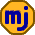## Wednesday, September 1, 2010

### Understanding Correlation

The idea of correlation can be confusing. Most of us have an idea of what seems correlated when we look at a stock chart, but this everyday idea of correlation can be very different from mathematical correlation.

Consider the following hypothetical chart of two stock indexes.

What would you guess is the correlation is between stocks indexes in this chart? Maybe 70% or 80%? They seem to move together quite a bit. When we focus on 1-year periods, the answer is a correlation of -0.2%! That's right – essentially no correlation.

However, if we look at rolling 5-year periods, the correlation is 88%. So, we get a completely different picture if we look at 1-year returns versus 5-year returns.

This discussion of 1-year and 5-year correlations can be confusing. After all, aren't we talking about 30 years here? Mathematical correlation in this context is about comparing two strings of numbers. We can make these two strings of numbers be 30 numbers long and consist of 1-year growth values, or we can make the strings 26 numbers long and consist of rolling 5-year growth values.

There is always a way to look at the numbers to get the result you want. As the saying goes, there are lies, damned lies, and statistics.

1.Wow. That's an eye-opener.

2.I don't think that means correlation is a useless investment statistic. What happens when you have a portfolio of the two stocks in equal proportions and rebalance annually? I bet it's a smoother curve than either stock's and the return will be higher too. The reason we are interested in correlation is to manage a portfolio by taking advantage of rebalancing over shorter time periods than 5 years.

If we took actual 25 year rolling returns I bet every investment type / asset class would have correlation near +1 since over that long a time, just about everything goes up.

But your point is well taken, Michael, it is essential to understand how correlation works and how it is useful.

3.@Canadian Investor: You're right. Correlation definitely has its uses. My main point is that if two quantities are uncorrelated, it doesn't mean that they have no relationship to each other.

For example, if you choose a number uniformly at random between -10 and +10 this number is uncorrelated with its own square. But, these two quantities are obviously related in that you can calculate the second from the first.